# Unitriangular matrix group of degree three

(diff) ← Older revision | Latest revision (diff) | Newer revision → (diff)

## Definition

Note that the notation$U(3,\_)$ used here is sometimes also used for the unitary group, a totally different type of group. Thus, we here stick to the notation$UT(3,\_)$ which is less ambiguous.

### Over a unital ring

Suppose$R$ is a unital ring. The unitriangular matrix group of degree three over$R$, denoted$U(3,R)$,$UT(3,R)$, or$UL(3,R)$, is defined as the unitriangular matrix group of$3 \times 3$ matrices over$R$. Explicitly, it can be described as the group of upper triangular matrices with 1s on the diagonal, and entries over$R$ (with the group operation being matrix multiplication).

Each such matrix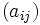$(a_{ij})$ can be described by the three entries$a_{12}, a_{13}, a_{23}$, each of which varies freely over$R$. The matrix looks like:$\begin{pmatrix} 1 & a_{12} & a_{13} \\ 0 & 1 & a_{23}\\ 0 & 0 & 1\end{pmatrix}$

In other words, the group itself is given as the following set under matrix multiplication:$\left \{\begin{pmatrix} 1 & a_{12} & a_{13} \\ 0 & 1 & a_{23}\\ 0 & 0 & 1\end{pmatrix} \mid a_{12},a_{13},a_{23} \in R \right \}$

The multiplication of matrices$A = (a_{ij})$ and$B = (b_{ij})$ gives the matrix$C = (c_{ij})$ where:

•$c_{12} = a_{12} + b_{12}$
•$c_{13} = a_{13} + b_{13} + a_{12}b_{23}$
•$c_{23} = a_{23} + b_{23}$

The identity element is the identity matrix.

The inverse of a matrix$A = (a_{ij})$ is the matrix$M = (m_{ij})$ where:

•$m_{12} = -a_{12}$
•$m_{13} = -a_{13} + a_{12}a_{23}$
•$m_{23} = -a_{23}$

### In coordinate form

We may define the group as the set of ordered triples$(a_{12},a_{13},a_{23})$ over the ring$R$ (the coordinates are allowed to repeat), with the multiplication law, identity element, and inverse operation given by: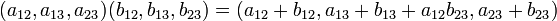$(a_{12},a_{13},a_{23}) (b_{12},b_{13},b_{23}) = (a_{12} + b_{12},a_{13} + b_{13} + a_{12}b_{23}, a_{23} + b_{23})$$\mbox{Identity element} = (0,0,0)$$(a_{12},a_{13},a_{23})^{-1} = (-a_{12}, -a_{13} + a_{12}a_{23}, -a_{23})$

The matrix corresponding to triple$(a_{12},a_{13},a_{23})$ is:$\begin{pmatrix} 1 & a_{12} & a_{13}\\ 0 & 1 & a_{23}\\ 0 & 0 & 1\\ \end{pmatrix}$

This definition clearly matches the earlier definition, based on the rules of matrix multiplication.

### Definition by presentation

The presentation given here is based on the presentation for unitriangular matrix group of degree three. We denote by$e_{ij}(\lambda)$ the matrix with 1s on the diagonal,$\lambda$ in the$(i,j)^{th}$ entry, and zeros elsewhere. Then:

• The following is a generating set: For every element$\lambda \in R$ and for$1 \le i , include the element$e_{ij}(\lambda)$. Since$n = 3$, this means that for every$\lambda$, we have generators$e_{12}(\lambda)$,$e_{13}(\lambda)$, and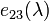$e_{23}(\lambda)$.
• We can get a presentation of the group using this generating set, by including the following relations:
Relation type All relations of this type (i.e., we plug in all possible$(i,j)$ values)$e_{ij}(\lambda)e_{ij}(\mu) = e_{ij}(\lambda + \mu)$ (note that this in particular implies that$e_{ij}(0)$ is the identity element for all$i,j$).$e_{12}(\lambda)e_{12}(\mu) = e_{12}(\lambda + \mu)$$e_{13}(\lambda)e_{13}(\mu) = e_{13}(\lambda + \mu)$$e_{23}(\lambda)e_{23}(\mu) = e_{23}(\lambda + \mu)$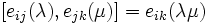$[e_{ij}(\lambda),e_{jk}(\mu)] = e_{ik}(\lambda \mu)$ (note that$\lambda$ and$\mu$ are allowed to be equal or different).$[e_{12}(\lambda),e_{23}(\mu)] = e_{13}(\mu)$$[e_{ij}(\lambda),e_{kl}(\mu)] = 1$ (i.e., is the identity element) for$i \ne l, j \ne k$. (note that$\lambda$ and$\mu$ are allowed to be equal or different).$[e_{12}(\lambda),e_{12}(\mu)] = 1$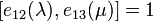$[e_{12}(\lambda),e_{13}(\mu)] = 1$$[e_{13}(\lambda),e_{12}(\mu)] = 1$$[e_{13}(\lambda),e_{13}(\mu)] = 1$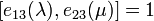$[e_{13}(\lambda),e_{23}(\mu)] = 1$$[e_{23}(\lambda),e_{13}(\mu)] = 1$$[e_{23}(\lambda),e_{23}(\mu)] = 1$

Note that this presentation can be trimmed quite a bit. In fact, if$A$ is a generating set for the additive group of$R$, the set:$\{ e_{12}(\lambda) \mid \lambda \in A \} \cup \{ e_{23}(\lambda) \mid \lambda \in A \}$

is a generating set for$UT(3,R)$, and we can work out a presentation in terms of this generating set.

### Over a field

The same definition as for a unital ring applies to the case where we are working over a field. For a field$K$, the group$U(3,K)$ (or$UT(3,K)$) is defined as: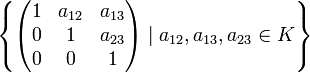$\left \{ \begin{pmatrix} 1 & a_{12} & a_{13} \\ 0 & 1 & a_{23}\\ 0 & 0 & 1\end{pmatrix} \mid a_{12}, a_{13}, a_{23} \in K \right \}$

### Over a finite field

For a prime power$q$, the unitriangular matrix group of degree three, denoted$UT(3,q)$ or$UL(3,q)$, is defined as the unitriangular matrix group of degree three over the unique finite field of$q$ elements. It can also be defined in other equivalent ways, where$p$ is the characteristic of the field (i.e., the underlying prime whose power is$q$:

1. It is one of the$p$-Sylow subgroups of the general linear group of degree three over$\mathbb{F}_q$, i.e.,$GL(3,q)$.
2. It is one of the$p$-Sylow subgroups of the special linear group of degree three over$\mathbb{F}_q$, i.e.,$SL(3,q)$.
3. It is isomorphic to the$p$-Sylow subgroups of the projective general linear group of degree three over$\mathbb{F}_q$, i.e.,$PGL(3,q)$.
4. It is isomorphic to the$p$-Sylow subgroups of the projective special linear group of degree three over$\mathbb{F}_q$, i.e.,$PSL(3,q)$.

### Over a finite prime field

The unitriangular matrix group of degree three over a finite prime field$\mathbb{F}_p$ is an important non-abelian group of order$p^3$. For more on this group, see unitriangular matrix group:UT(3,p).

### Upper triangular versus lower triangular

The unitriangular matrix group of degree three can also be defined as the group of lower triangular matrices with 1s on the diagonal. This is because the conjugation by the antidiagonal permutation conjugates the subgroup of upper triangular matrices to the subgroup of lower triangular matrices, and the eigenvalues remain the same.